SLOS708G April   2012  – December 2017

PRODUCTION DATA.

1. Features
2. Applications
3. Description
4. Revision History
5. Pin Configuration and Functions
6. Specifications
7. Detailed Description
1. 7.1 Overview
2. 7.2 Functional Block Diagram
3. 7.3 Feature Description
4. 7.4 Device Functional Modes
8. Application and Implementation
1. 8.1 Application Information
2. 8.2 Typical Application
9. Power Supply Recommendations
10. 10Layout
11. 11Device and Documentation Support
12. 12Mechanical, Packaging, and Orderable Information

6 Specifications

6.1 Absolute Maximum Ratings

over operating free-air temperature range (unless otherwise noted)(1)
MIN MAX UNIT
Supply voltage, VCC PVCC, AVCC –0.3 30 V
Input voltage, VI INPL, INNL, INPR, INNR –0.3 6.3 V
PLIMIT, GAIN / SLV, SYNC –0.3 GVDD+0.3 V
AM0, AM1, AM2, MUTE, SDZ, MODSEL –0.3 PVCC+0.3 V
Slew rate, maximum(2) AM0, AM1, AM2, MUTE, SDZ, MODSEL 10 V/ms
Operating free-air temperature, TA –40 85 °C
Operating junction temperature , TJ –40 150 °C
Storage temperature, Tstg –40 125 °C
Stresses beyond those listed under absolute maximum ratings may cause permanent damage to the device. These are stress ratings only, and functional operation of the device at these or any other conditions beyond those indicated under recommended operating conditions is not implied. Exposure to absolute-maximum-rated conditions for extended periods may affect device reliability.
100 kΩ series resistor is needed if maximum slew rate is exceeded.

6.2 ESD Ratings

VALUE UNIT
V(ESD) Electrostatic discharge Human-body model (HBM), per ANSI/ESDA/JEDEC JS-001(1) ±2000 V
Charged-device model (CDM), per JEDEC specification JESD22-C101(2) ±500
JEDEC document JEP155 states that 500-V HBM allows safe manufacturing with a standard ESD control process.
JEDEC document JEP157 states that 250-V CDM allows safe manufacturing with a standard ESD control process. .

6.3 Recommended Operating Conditions

over operating free-air temperature range (unless otherwise noted)
MIN NOM MAX UNIT
VCC Supply voltage PVCC, AVCC 4.5 26 V
VIH High-level input voltage AM0, AM1, AM2, MUTE, SDZ, SYNC, MODSEL 2 V
VIL Low-level input voltage AM0, AM1, AM2, MUTE, SDZ, SYNC, MODSEL 0.8 V
VOL Low-level output voltage FAULTZ, RPULL-UP = 100 kΩ, PVCC = 26 V 0.8 V
IIH High-level input current AM0, AM1, AM2, MUTE, SDZ, MODSEL (VI = 2 V, VCC = 18 V) 50 µA
RL(BTL) Minimum load Impedance Output filter: L = 10 µH, C = 680 nF TPA3116D2, TPA3118D2 3.2 4 Ω
TPA3130D2 5.6 8
RL(PBTL) Output filter: L = 10 µH, C = 1 µF TPA3116D2, TPA3118D2 1.6
TPA3130D2 3.2 4
Lo Output-filter Inductance Minimum output filter inductance under short-circuit condition 1 µH

6.4 Thermal Information

THERMAL METRIC(1) TPA3130D2 TPA3118D2 TPA3116D2 UNIT
32 PINS 32 PINS 32 PINS
RθJA Junction-to-ambient thermal resistance 36 22 14 °C/W
ψJT Junction-to-top characterization parameter 0.4 0.3 1.2
ψJB Junction-to-board characterization parameter 5.9 4.7 5.7
For the PCB layout please see the TPA3130D2EVM user guide.
For the PCB layout please see the TPA3118D2EVM user guide.
The heat sink drawing used for the thermal model data are shown in the application section, size: 14mm wide, 50mm long, 25mm high.

6.5 DC Electrical Characteristics

TA = 25°C, AVCC = PVCC = 12 V to 24 V, RL = 4 Ω (unless otherwise noted)
PARAMETER TEST CONDITIONS MIN TYP MAX UNIT
| VOS | Class-D output offset voltage (measured differentially) VI = 0 V, Gain = 36 dB 1.5 15 mV
ICC Quiescent supply current SDZ = 2 V, No load or filter, PVCC = 12 V 20 35 mA
SDZ = 2 V, No load or filter, PVCC = 24 V 32 50
ICC(SD) Quiescent supply current in shutdown mode SDZ = 0.8 V, No load or filter, PVCC = 12 V <50 µA
SDZ = 0.8 V, No load or filter, PVCC = 24 V 50 400
rDS(on) Drain-source on-state resistance, measured pin to pin PVCC = 21 V, Iout = 500 mA, TJ = 25°C 120
G Gain (BTL) R1 = 5.6 kΩ, R2 = Open 19 20 21 dB
R1 = 20 kΩ, R2 = 100 kΩ 25 26 27
R1 = 39 kΩ, R2 = 100 kΩ 31 32 33 dB
R1 = 47 kΩ, R2 = 75 kΩ 35 36 37
G Gain (SLV) R1 = 51 kΩ, R2 = 51 kΩ 19 20 21 dB
R1 = 75 kΩ, R2 = 47 kΩ 25 26 27
R1 = 100 kΩ, R2 = 39 kΩ 31 32 33 dB
R1 = 100 kΩ, R2 = 16 kΩ 35 36 37
ton Turn-on time SDZ = 2 V 10 ms
tOFF Turn-off time SDZ = 0.8 V 2 µs
GVDD Gate drive supply IGVDD < 200 µA 6.4 6.9 7.4 V
VO Output voltage maximum under PLIMIT control V(PLIMIT) = 2 V; VI = 1 Vrms 6.75 7.90 8.75 V

6.6 AC Electrical Characteristics

TA = 25°C, AVCC = PVCC = 12 V to 24 V, RL = 4 Ω (unless otherwise noted)
PARAMETER TEST CONDITIONS MIN TYP MAX UNIT
KSVR Power supply ripple rejection 200 mVPP ripple at 1 kHz, Gain = 20 dB, Inputs AC-coupled to GND –70 dB
PO Continuous output power THD+N = 10%, f = 1 kHz, PVCC = 14.4 V 25 W
THD+N = 10%, f = 1 kHz, PVCC = 21 V 50
THD+N Total harmonic distortion + noise VCC = 21 V, f = 1 kHz, PO = 25 W (half-power) 0.1%
Vn Output integrated noise 20 Hz to 22 kHz, A-weighted filter, Gain = 20 dB 65 µV
–80 dBV
Crosstalk VO = 1 Vrms, Gain = 20 dB, f = 1 kHz –100 dB
SNR Signal-to-noise ratio Maximum output at THD+N < 1%, f = 1 kHz, Gain = 20 dB, A-weighted 102 dB
fOSC Oscillator frequency AM2=0, AM1=0, AM0=0 376 400 424 kHz
AM2=0, AM1=0, AM0=1 470 500 530
AM2=0, AM1=1, AM0=0 564 600 636
AM2=0, AM1=1, AM0=1 940 1000 1060
AM2=1, AM1=0, AM0=0 1128 1200 1278
AM2=1, AM1=0, AM0=1 Reserved
AM2=1, AM1=1, AM0=0
AM2=1, AM1=1, AM0=1
Thermal trip point 150+ °C
Thermal hysteresis 15 °C
Over current trip point TPA3130D2 4.5 A
TPA3118D2, TPA3116D2 7.5

6.7 Typical Characteristics

fs = 400 kHz, BD Mode (unless otherwise noted)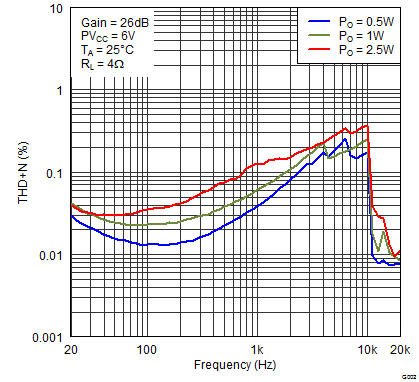Figure 1. Total Harmonic Distortion + Noise (BTL) vs Frequency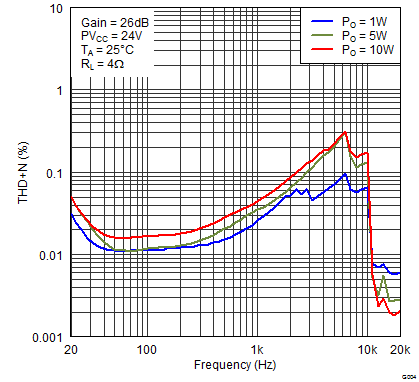Figure 3. Total Harmonic Distortion + Noise (BTL) vs Frequency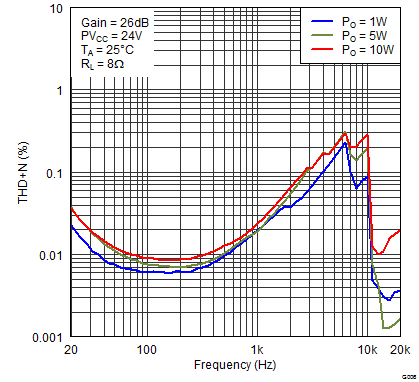Figure 5. Total Harmonic Distortion + Noise (BTL) vs Frequency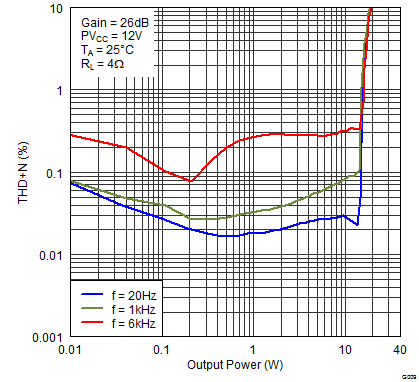Figure 7. Total Harmonic Distortion + Noise (BTL) vs Output PowerFigure 9. Total Harmonic Distortion + Noise (BTL) vs Output Power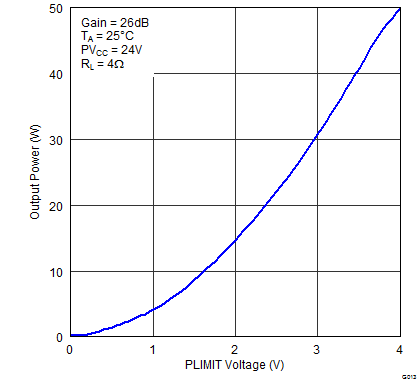Figure 11. Output Power (BTL) vs Plimit Voltage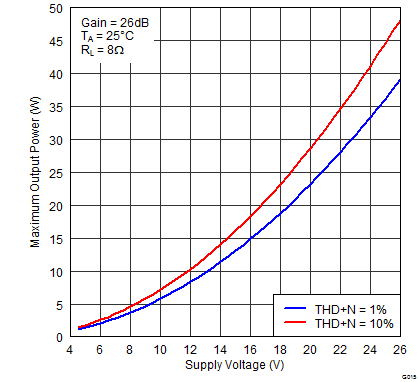Figure 13. Maximum Output Power (BTL) vs Supply VoltageFigure 15. Power Efficiency (BTL) vs Output PowerFigure 17. Crosstalk vs Frequency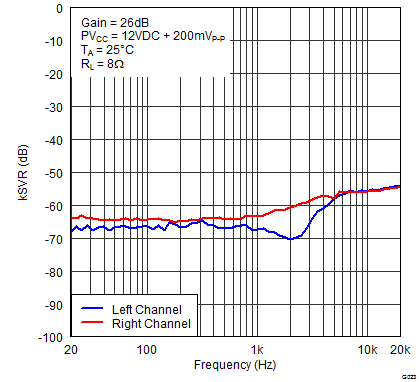Figure 19. Supply Ripple Rejection Ratio (BTL) vs Frequency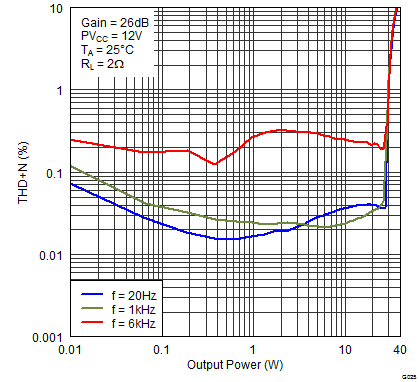Figure 21. Total Harmonic Distortion + Noise (PBTL) vs Output PowerFigure 23. Power Efficiency (PBTL) vs Output Power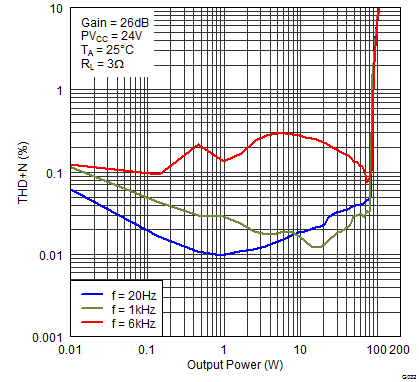Figure 25. Total Harmonic Distortion + Noise (PBTL) vs Output PowerFigure 2. Total Harmonic Distortion + Noise (BTL) vs Frequency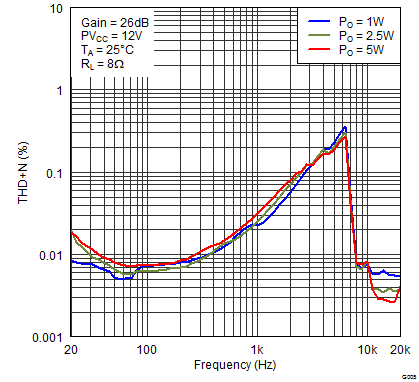Figure 4. Total Harmonic Distortion + Noise (BTL) vs Frequency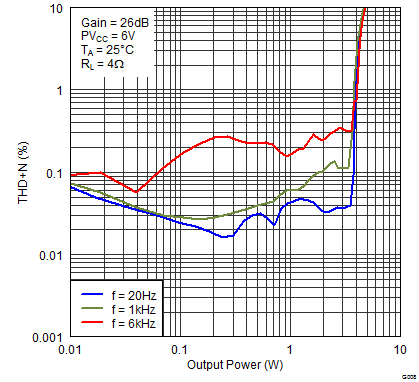Figure 6. Total Harmonic Distortion + Noise (BTL) vs Output Power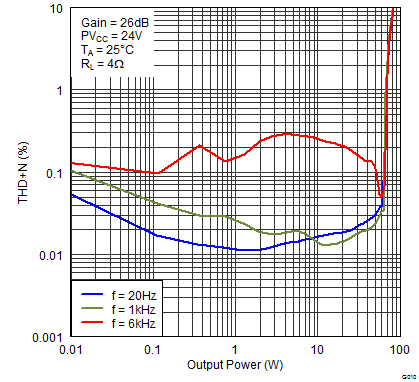Figure 8. Total Harmonic Distortion + Noise (BTL) vs Output Power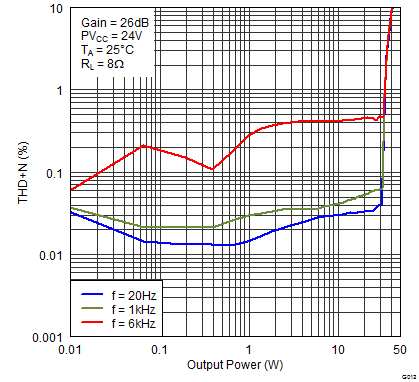Figure 10. Total Harmonic Distortion + Noise (BTL) vs Output Power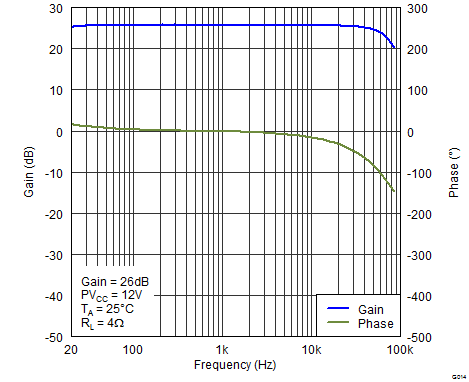Figure 12. Gain/Phase (BTL) vs Frequency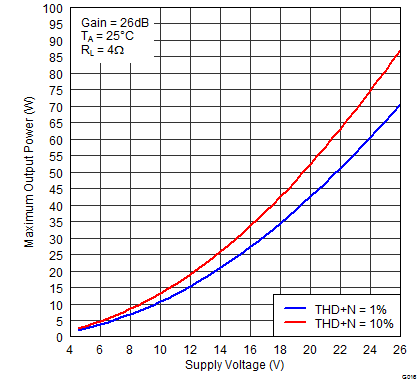Figure 14. Maximum Output Power (BTL) vs Supply Voltage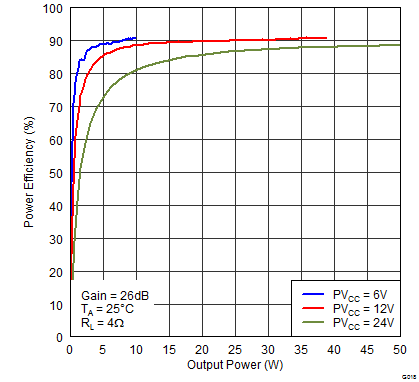Figure 16. Power Efficiency (BTL) vs Output PowerFigure 18. Crosstalk vs Frequency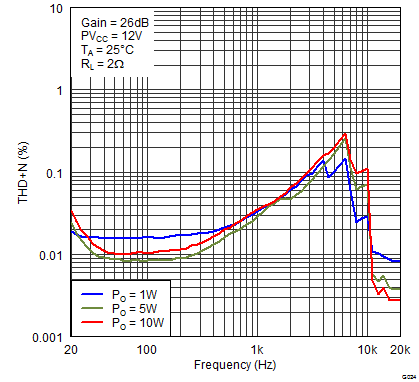Figure 20. Total Harmonic Distortion + Noise (PBTL) vs Frequency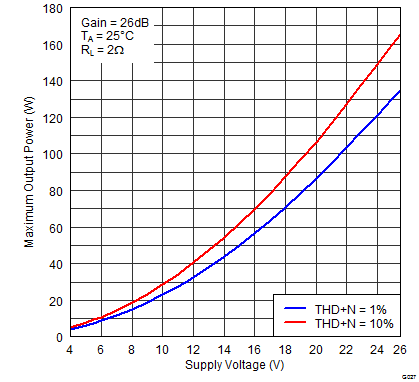Figure 22. Maximum Output Power (PBTL) vs Supply VoltageFigure 24. Supply Ripple Rejection Ratio (PBTL) vs Frequency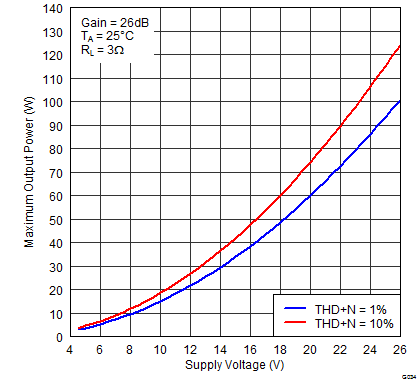Figure 26. Maximum Output Power (PBTL) vs Supply Voltage